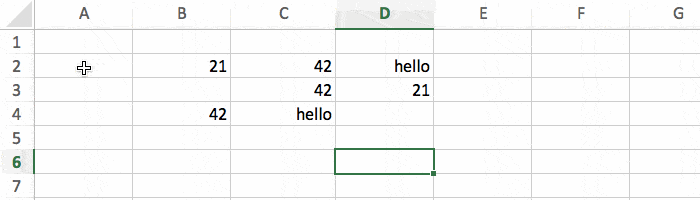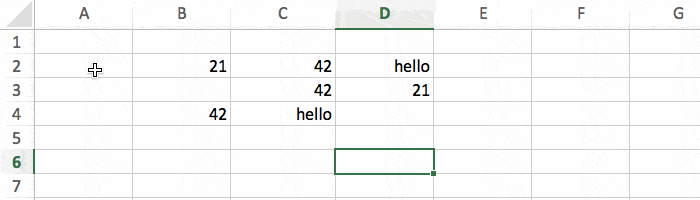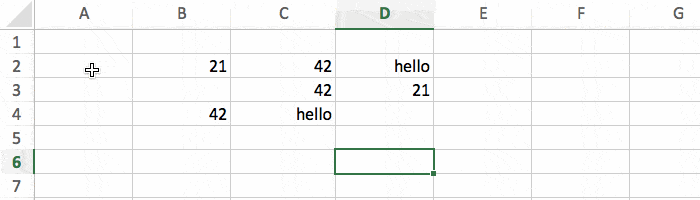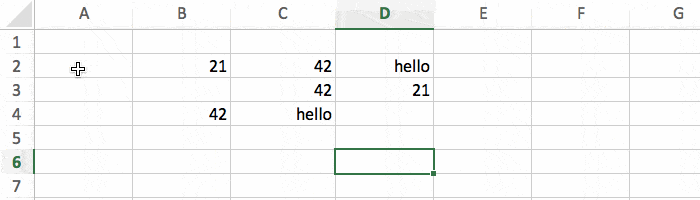# Excel: How and When To Use The Four COUNT Functions

A lot of people know they can use COUNT to count cells in Microsoft Excel. But there are actually 4 different COUNT functions available, and you might not know their subtle differences. We are going to cover them in detail in this short tutorial.

## The COUNT Function

Let's start with the most basic function: COUNT. It's important to know that it only counts cells containing numbers. All other cells will be ignored (texts, dates, blanks).

`=COUNT(range)`

Here's how it works with a simple example.There are 5 numbers in the range, and the result is 5. As expected!

## The COUNTA Function

If you want to count cells with any type of data (and not just numbers), simply replace COUNT by COUNTA. This will count all cells that are not blank (numbers, texts, dates).There are 5 numbers and 2 texts, 5+2 = 7.

## The COUNTBLANK Function

What if you want to do the opposite: only count the blank cells? Then you should use COUNTBLANK.There are 2 blank cells, so the result is correct.

## The COUNTIF Function

For more advanced ways to count, you'll have to use COUNTIF. This is basically a combination between the 2 functions COUNT and IF.

`=COUNTIF(range, condition)`

It will count the cells in the range only if they meet the condition.

For our example, if you want to count only the values that are higher than 30, use this:

`=COUNTIF(B2:D4, ">30")`

You have to put quotes above the condition, otherwise it won't work.There are only 3 cells above 30, and the result is 3. So It seems to be working :-)

## Bonus

What if you want to count every cell in a range? Both the ones with content and the ones that are empty? For this to work you have to use 2 functions at the same time:

`=COUNTA(range) + COUNTBLANK(range)`

There's no built in function to do this, so that's the only solution.

## Conclusion

You can see that for something as simple as "count the number of cells in a range", you have lots of options to choose from. So make sure you understand the differences between all these functions:

• `COUNT(range)`, count only numbers.
• `COUNTA(range)`, count all except blank.
• `COUNTBLANK(range)`, count only blank.
• `COUNTIF(range, condition)`, count if match the condition.